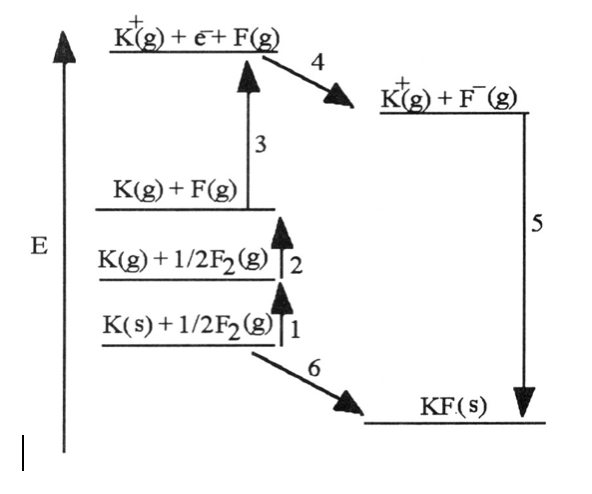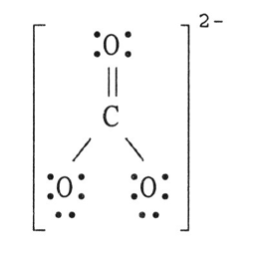# Chemistry: Ch:8 Study Packet Flashcards

Set Details Share
created 5 years ago by jessjess2601
4,503 views
updated 5 years ago by jessjess2601
Subjects:
chemistry, science
Page to share:
Embed this setcancel
COPY
code changes based on your size selection
Size:
X

1

1) Which of the following has eight valence electrons?

A) Ti4+

B) Kr

C) Cl-

D) Na+

E) all of the above

E) all of the above

2

2) Which of the following does not have eight valence electrons?

A) Ca+

B) Rb+

C) Xe

D) Br-

E) All of the above have eight valence electrons.

A) Ca+

3

3) Lattice energy is ________.

A) the energy required to convert a mole of ionic solid into its constituent ions in the gas phase

B) the energy given off when gaseous ions combine to form one mole of an ionic solid

C) the energy required to produce one mole of an ionic compound from its constituent elements in their standard states

D) the sum of ionization energies of the components in an ionic solid

E) the sum of electron affinities of the components in an ionic solid

A) the energy required to convert a mole of ionic solid into its constituent ions in the gas phase

4

4) In ionic bond formation, the lattice energy of ions ________ as the magnitude of the ion charges _______ and the radii ________.

A) increases, decrease, increase

B) increases, increase, increase

C) decreases, increase, increase

D) increases, increase, decrease

E) increases, decrease, decrease

D) increases, increase, decrease

55) Which energy change corresponds to the electron affinity of fluorine?

A) 2

B) 5

C) 4

D) 1

E) 6

C) 4

66) Which energy change corresponds to the first ionization energy of potassium?

A) 2

B) 5

C) 4

D) 3

E) 6

D) 3

7

7) Using the Born-Haber cycle, the ΔH°f of KBr is equal to ________.

A) ΔH°f [K (g)] + ΔH°f [Br (g)] + I1(K) + E(Br) + ΔHlattice

B) ΔH°f [K (g)] - ΔH°f [Br (g)] - I1(K) - E(Br) - ΔHlattice

C) ΔH°f [K (g)] - ΔH°f [Br (g)] + I1(K) - E(Br) + ΔHlattice

D) ΔH°f [K (g)] + ΔH°f [Br (g)] - I1 - E(Br) + ΔHlattice

E) ΔH°f [K (g)] + ΔH°f [Br (g)] + I1(K) + E(Br) - ΔHlattice

E) ΔH°f [K (g)] + ΔH°f [Br (g)] + I1(K) + E(Br) - ΔHlattice

8

8) The type of compound that is most likely to contain a covalent bond is ________.

A) one that is composed of a metal from the far left of the periodic table and a nonmetal from the far right of the periodic table

B) a solid metal

C) one that is composed of only nonmetals

D) held together by the electrostatic forces between oppositely charged ions

E) There is no general rule to predict covalency in bonds.

C) one that is composed of only nonmetals

9

9) In which of the molecules below is the carbon-carbon distance the shortest?

A) H2C CH2

B) H-C≡C-H

C) H3C—CH3

D) H2C C CH2

E) H3C—CH2—CH3

B) H-C≡C-H

10

10) Of the molecules below, the bond in ________ is the most polar.

A) HBr

B) HI

C) HCl

D) HF

E) H2

D) HF

11

11) Which of the following has the bonds correctly arranged in order of increasing polarity?

A) Be—F, Mg—F, N—F, O—F

B) O—F, N—F, Be—F, Mg—F

C) O—F, Be—F, Mg—F, N—F

D) N—F, Be—F, Mg—F, O—F

E) Mg—F, Be—F, N—F, O—F

B) O—F, N—F, Be—F, Mg—F

12

12) The bond length in an HI molecule is 1.61 Å and the measured dipole moment is 0.44 D. What is the magnitude (in units of e) of the negative charge on I in HI?

(1 debye = 3.34 × 10-30 coulomb-meters; e = 1.6 × 10-19 coulombs)

A) 1.6 × 10-19

B) 0.057

C) 9.1

D) 1

E) 0.22

B) 0.057

13

13) The Lewis structure of N2H2 shows ________.

A) a nitrogen-nitrogen triple bond

B) a nitrogen-nitrogen single bond

C) each nitrogen has one nonbonding electron pair

D) each nitrogen has two nonbonding electron pairs

E) each hydrogen has one nonbonding electron pair

C) each nitrogen has one nonbonding electron pair

14

14) The Lewis structure of the CO32- ion is ________.A.

15

15) In the nitrite ion (NO2-), ________.

A) both bonds are single bonds

B) both bonds are double bonds

C) one bond is a double bond and the other is a single bond

D) both bonds are the same

E) there are 20 valence electrons

C) one bond is a double bond and the other is a single bond

16

16) Resonance structures differ by ________.

A) number and placement of electrons

B) number of electrons only

C) placement of atoms only

D) number of atoms only

E) placement of electrons only

E) placement of electrons only

17

17) The oxidation number of iron in Fe2O3 is ________.

A) -2

B) +1

C) +3

D) +2

E) -3

C) +3

18

18) To convert from one resonance structure to another, ________.

A) only atoms can be moved

B) electrons and atoms can both be moved

C) only electrons can be moved

D) neither electrons nor atoms can be moved

C) only electrons can be moved

19

19) For resonance forms of a molecule or ion, ________.

A) one always corresponds to the observed structure

B) all the resonance structures are observed in various proportions

C) the observed structure is an average of the resonance forms

D) the same atoms need not be bonded to each other in all resonance forms

E) there cannot be more than two resonance structures for a given species

C) the observed structure is an average of the resonance forms

20

For the questions that follow, consider the BEST Lewis structures of the following oxyanions:

(i)NO2- (ii)NO3- (iii)SO32- (iv)SO42- (v)BrO3-

20) There can be four equivalent best resonance structures of ________.

A) (i)

B) (ii)

C) (iii)

D) (iv)

E) (v)

D) (iv)

21

For the questions that follow, consider the BEST Lewis structures of the following oxyanions:

(i)NO2- (ii)NO3- (iii)SO32- (iv)SO42- (v)BrO3-

21) In which of the ions do all X-O bonds (X indicates the central atom) have the same length?

A) none

B) all

C) (i) and (ii)

D) (iii) and (v)

E) (iii), (iv), and (v)

B) all

22

22) A valid Lewis structure of ________ cannot be drawn without violating the octet rule.

A) NF3

B) IF3

C) PF3

D) SbF3

E) SO42-

B) IF3

23

23) Based on the octet rule, boron will most likely form a ________ ion.

A) B3-

B) B+

C) B3+

D) B2+

E) B2-

C) B3+

24

24) Which of the following does not have eight valence electrons?

A) Cl-

B) Xe

C) Ti+4

D) Rb+

E) Sr+

E) Sr+

25

25) A valid Lewis structure of ________ cannot be drawn without violating the octet rule.

A) PO43-

B) SiF4

C) CF4

D) SeF4

E) NF3

D) SeF4

26

26) The central atom in ________ does not violate the octet rule.

A) SF4

B) KrF2

C) CF4

D) XeF4

E) ICl4-

C) CF4

27

27) A valid Lewis structure of ________ cannot be drawn without violating the octet rule.

A) ClF3

B) PCl3

C) SO3

D) CCl4

E) CO2

A) ClF3

28

28) A valid Lewis structure of ________ cannot be drawn without violating the octet rule.

A) NI3

B) SO2

C) ICl5

D) SiF4

E) CO2

C) ICl5

29

29) A valid Lewis structure of ________ cannot be drawn without violating the octet rule.

A) NF3

B) BeH2

C) SO2

D) CF4

E) SO32-

B) BeH2

30

30) Why don't we draw double bonds between the Be atom and the Cl atoms in BeCl2?

A) That would give positive formal charges to the chlorine atoms and a negative formal charge to the beryllium atom.

B) There aren't enough electrons.

C) That would result in more than eight electrons around beryllium.

D) That would result in more than eight electrons around each chlorine atom.

E) That would result in the formal charges not adding up to zero.

A) That would give positive formal charges to the chlorine atoms and a negative formal charge to the beryllium atom.

31

31) Which atom can accommodate an octet of electrons, but doesn't necessarily have to accommodate an octet?

A) N

B) C

C) H

D) O

E) B

E) B

32

32) Bond enthalpy is ________.

A) always positive

B) always negative

C) sometimes positive, sometimes negative

D) always zero

E) unpredictable

A) always positive

33

33) Given that the average bond energies for C-H and C-Br bonds are 413 and 276 kJ/mol, respectively, the heat of atomization of bromoform (CHBr3) is ________ kJ/mol.

A) 1241

B) 689

C) -689

D) 1378

E) -1378

A) 1241

34

34) Of the bonds C-C, C C, and C≡C, the C-C bond is ________.

A) strongest/shortest

B) strongest/longest

C) weakest/longest

D) weakest/shortest

E) intermediate in both strength and length

C) weakest/longest

35

35) Of the bonds C-N, C N, and C≡N, the C-N bond is ________.

A) strongest/shortest

B) strongest/longest

C) weakest/shortest

D) weakest/longest

E) intermediate in both strength and length

D) weakest/longest

36

36) As the number of covalent bonds between two atoms increases, the distance between the atoms ________ and the strength of the bond between them ________.

A) increases, increases

B) decreases, decreases

C) increases, decreases

D) decreases, increases

E) is unpredictable

D) decreases, increases

37

37) Of the possible bonds between carbon atoms (single, double, and triple), ________.

A) a triple bond is longer than a single bond

B) a double bond is stronger than a triple bond

C) a single bond is stronger than a triple bond

D) a double bond is longer than a triple bond

E) a single bond is stronger than a double bond

D) a double bond is longer than a triple bond

38

38) Most explosives are compounds that decompose rapidly to produce ________ products and a great deal of ________.

A) gaseous, gases

B) liquid, heat

C) soluble, heat

D) solid, gas

E) gaseous, heat

E) gaseous, heat

39

39) Dynamite consists of nitroglycerine mixed with ________.

A) potassium nitrate

B) damp KOH

C) TNT

D) diatomaceous earth or cellulose

E) solid carbon

D) diatomaceous earth or cellulose

40

40) Dynamite ________.

A) was invented by Alfred Nobel

B) is made of nitroglycerine and an absorbent such as diatomaceous earth

C) is a much safer explosive than pure nitroglycerine

D) is an explosive

E) all of the above

E) all of the above

41

41) Based on the octet rule, magnesium most likely forms a ________ ion.

A) Mg2+

B) Mg2-

C) Mg6-

D) Mg6+

E) Mg-

A) Mg2+

42

42) The electron configuration of the phosphide ion (P3-) is ________.

A) [Ne]3s2

B) [Ne]3s23p1

C) [Ne]3s23p3

D) [Ne]3p2

E) [Ne]3s23p6

E) [Ne]3s23p6

43

43) The electron configuration of the sulfide ion (S2-) is ________.

A) [Ne]3s2

B) [Ne]3s23p1

C) [Ne]3s23p4

D) [Ne]3p2

E) [Ne]3s23p6

E) [Ne]3s23p6

44

44) The halogens, alkali metals, and alkaline earth metals have ________ valence electrons, respectively.

A) 7, 4, and 6

B) 1, 5, and 7

C) 8, 2, and 3

D) 7, 1, and 2

E) 2, 7, and 4

D) 7, 1, and 2

45

45) The only noble gas without eight valence electrons is ________.

A) Ar

B) Ne

C) He

D) Kr

E) All noble gases have eight valence electrons.

C) He